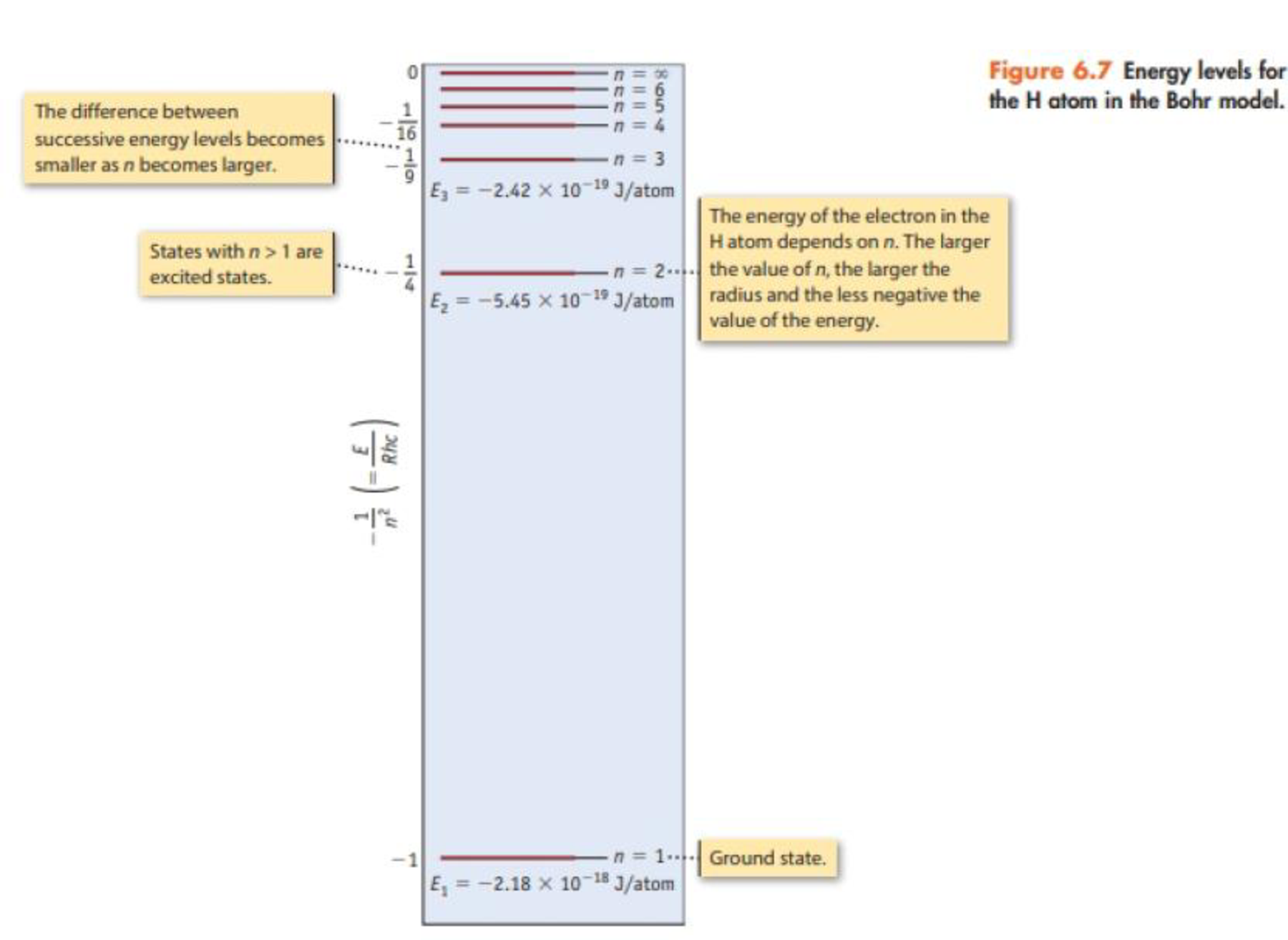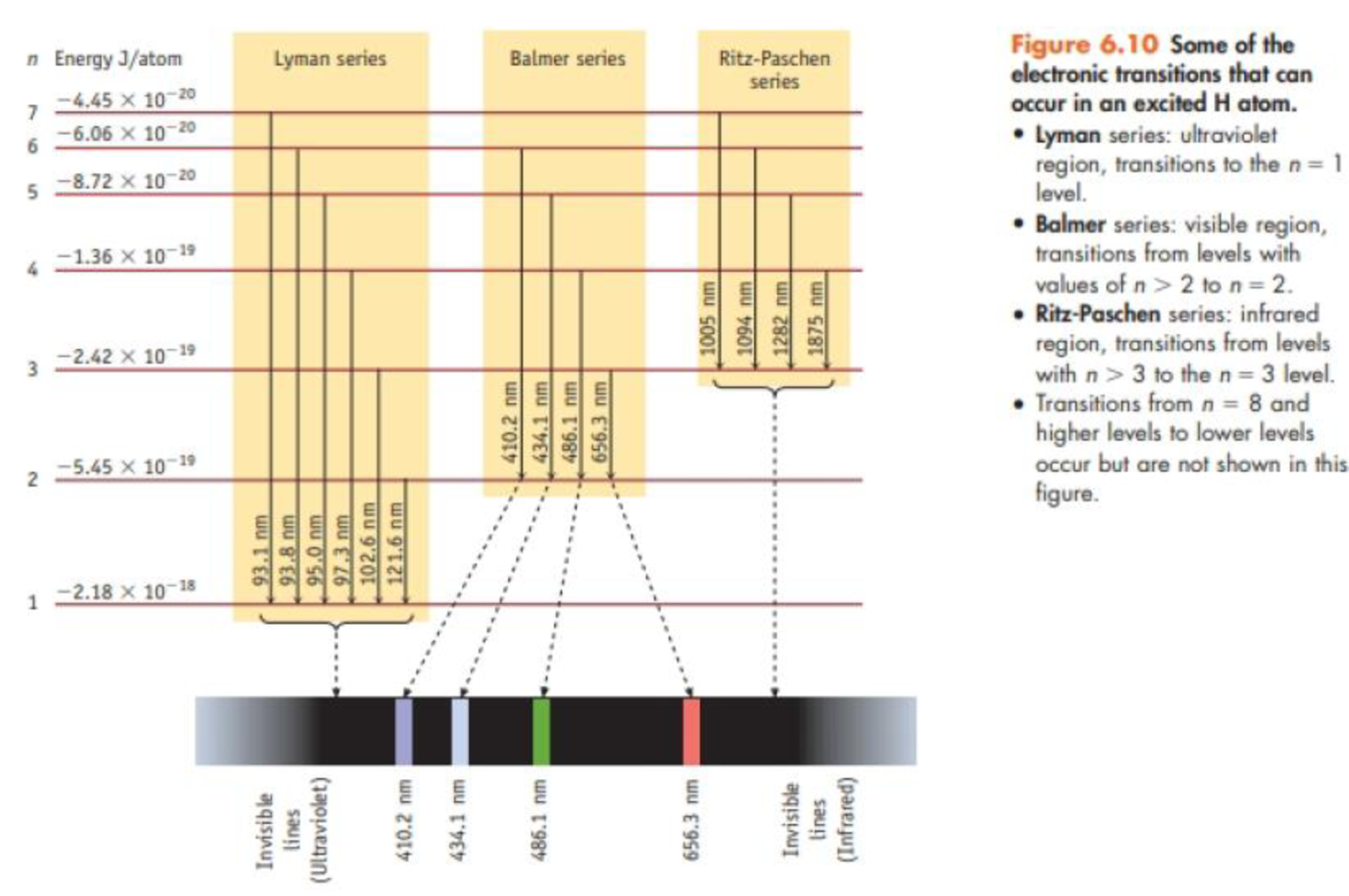Chapter 6, Problem 17PS

Chapter
Section
Textbook Problem

Consider only transitions involving the n = 1 through n = 5 energy levels for the H atom (see Figures 6.7 and 6.10). (a) How many emission lines are possible, considering only the five quantum levels? (b) Photons of the highest frequency are emitted in a transition from the level with n = ______ to a level with n = ______. (c) The emission line having the longest wavelength corresponds to a transition from the level with n = ______ to the level with n = _________.(a)

Interpretation Introduction

Interpretation The total number of emission lines, if only five quantum levels are considered has to be determined.

Concept introduction:

• Electronic transitions that take place in excited H atom is,

Lyman series: electronic transitions take place to the n=1 level and it is in ultraviolet region.

Balmer series: electronic transitions take place from n>2 to the n=2 level and it is in visible region.

Ritz-Paschen series: electronic transitions take place from n>3 to the n=3 level and it is in infrared region.

Brackett series: electronic transitions take place from n>4 to the n=4 level.

Pfund series: electronic transitions take place from n>5 to the n=5 level

Explanation

The highest electronic state is n=5 so the electronic transition from n=5,4,3,2 is possible to each of the corresponding lower electronic states

54,53,52

(b)

Interpretation Introduction

Interpretation The transition of the photons emitted with longest wavelength and highest frequency has to be determined.

Concept introduction:

• Electronic transitions that take place in excited H atom is,

Lyman series: electronic transitions take place to the n=1 level and it is in ultraviolet region.

Balmer series: electronic transitions take place from n>2 to the n=2 level and it is in visible region.

Ritz-Paschen series: electronic transitions take place from n>3 to the n=3 level and it is in infrared region.

Brackett series: electronic transitions take place from n>4 to the n=4 level.

Pfund series: electronic transitions take place from n>5 to the n=5 level

• Planck’s equation,

E=where, ν=frequencyλ=wavelength

• As the energy gap between two transition states increases the frequency of the radiation emitted also increases.

(c)

Interpretation Introduction

Interpretation The transition of the photons emitted with longest wavelength has to be determined.

Concept introduction:

• Electronic transitions that take place in excited H atom is,

Lyman series: electronic transitions take place to the n=1 level and it is in ultraviolet region.

Balmer series: electronic transitions take place from n>2 to the n=2 level and it is in visible region.

Ritz-Paschen series: electronic transitions take place from n>3 to the n=3 level and it is in infrared region.

Brackett series: electronic transitions take place from n>4 to the n=4 level.

Pfund series: electronic transitions take place from n>5 to the n=5 level

• Planck’s equation,

E=where, ν=frequencyλ=wavelength

• As the energy gap between two transition states increases the frequency of the radiation emitted also increases.

Still sussing out bartleby?

Check out a sample textbook solution.

See a sample solution

The Solution to Your Study Problems

Bartleby provides explanations to thousands of textbook problems written by our experts, many with advanced degrees!

Get Started